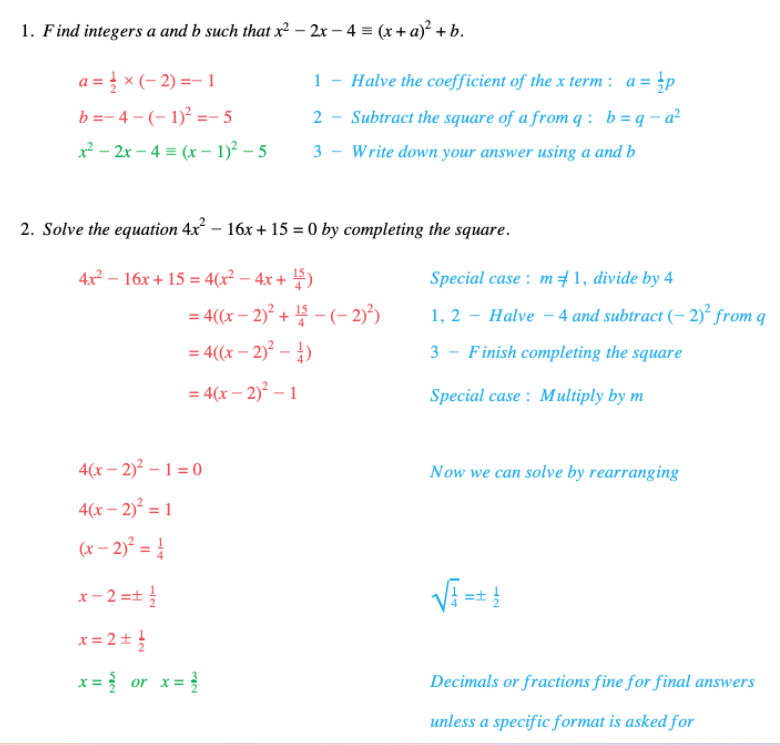# Edexcel IGCSE Maths 复习笔记 2.7.1 Completing the Square

Edexcel IGCSE Maths 复习笔记 2.7.1 Completing the Square

#### What is completing the square?

• “Completing the square” is something that can be done to a quadratic expression (to make it easier to work with or more useful in some way)
• It involves writing the quadratic expression x2 + px +q in the form (x + a)2 + b

#### Exam Tip

A question on this topic (see the question below) may use the Identity Symbol “≡” instead of an Equals Sign “=”.This tells you that what is on the left is exactly the same as what is on the right (no matter what value any letters take).You should never try to solve an identity like you might try to solve an equation.

#### Worked Example